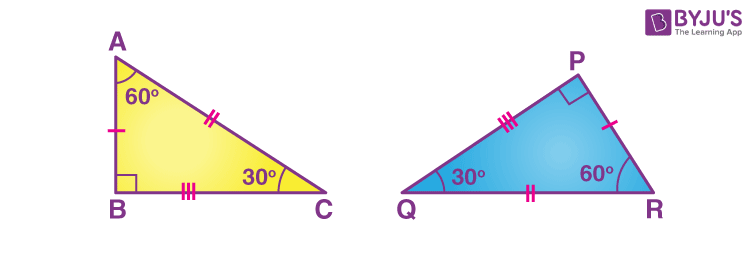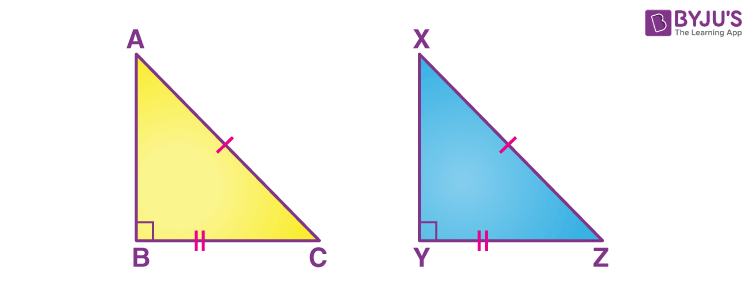# Right Triangle Congruence Theorem

A plane figure bounded by three finite line segments to form a closed figure is known as a triangle. A right-angled triangle is a special case of the triangle. In a right-angled triangle, one of the interior angles measures 90°. Two right triangles are said to be congruent if they are of the same shape and size. In other words, two right triangles are said to be congruent if the measure of the length of their corresponding sides and their corresponding angles is equal.

In fig. 1 given below, ∆ABC ≅ ∆RPQ since ∠A= ∠R, ∠C= ∠Q and ∠B= ∠P.

Also, AB = RP, BC = PQ and AC = QR.Now, we will discuss the different methods by which we can draw a triangle congruent to ∆ABC, which is right angled at B. This leads to a very important criterion known as the RHS congruence or right triangle congruence theorem.

According to the RHS Criterion:

Two right-angled triangles are said to be congruent to each other if the hypotenuse and one side of the right triangle are equal to the hypotenuse and the corresponding side of the other right-angled triangle.

## Right Triangle Congruence Theorem Example

Question: Consider two triangles, ΔABC and ΔXYZ such that: ∠B = ∠Y = 90°, AC = XZ and AB = XY. Prove that ΔABC ≅ ΔXYZ.Solution:

In ΔABC and ΔXYZ, it is given that AC = XZ, BC = YZ and ∠B = ∠Y. Thus, by the right triangle congruence theorem, since the hypotenuse and the corresponding bases of the given right triangles are equal, therefore, both these triangles are congruent to each other. Thus, ΔABC ≅ ΔXYZ.

Therefore by using the right triangle congruence theorem, we can easily deduce of two right triangles are congruent or not.

## Video Lesson on Congruent Triangles## Frequently Asked Questions – FAQs

Q1

### What is the Right Triangle Congruence Theorem?

The Right Triangle Congruence Theorem states that ‘’Two right triangles are said to be congruent if they are of the same shape and size’’.
Q2

### What are the four congruence theorems for right triangles?

Right Triangle Congruence
Leg-Leg Congruence. If the legs of a right triangle are congruent to the corresponding legs of another right triangle, then the triangles are congruent.
Hypotenuse-Angle Congruence.
Leg-Angle Congruence.
Hypotenuse-Leg Congruence.
Q3

### What are the 5 triangle congruence theorems?

The five theorems of congruent triangles are SSS, SAS, AAS, HL, and ASA.
SSS – side, side, and side.
SAS – side, angle, and side.
ASA – angle, side, and angle.
AAS – angle, angle, and side.
HL – hypotenuse and leg.
Q4

### What is LA Theorem?

LA theorem refers to leg-acute.
It states that if the leg and an acute angle of one right triangle are congruent to the corresponding leg and the acute angle of another right triangle, then the triangles are congruent.
Q5

### What is LL Theorem?

LL theorem is leg-leg.
It states that if the legs of one right triangle are congruent to the legs of another right triangle, then the triangles are congruent.